Next: The root-element Up: Elements of the Fib Previous: Set-element   Contents   Index

## Matrix element

The matrix element represents a matrix. The matrix element works similar to the set element, except that a number of counter variables is generated automatically.

Syntax:Description of the elements:

•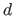: Number of dimensions of the matrix ()
•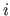: Number of values per set of values ()
•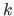: Number of sets, with which the variables are set (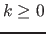)
•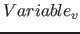: The variables, which the matrix element defines
•: This is the number of the domain for the matrix element. This information is optional, the default value is 0. If no matrix domain with that number exist, the matrix domain with the next smallest number is used. By using different, adapted for each matrix element, domains, the memory requirements for storing in the compressed Fib format can be optimized.
•: vector for the area for the (/ matrix size in) dimension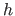•: Start value of the counter variable for the'th dimension
•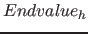: End value for the counter variable for the'th dimension
•withand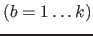: This are the to set values or variables, whith values to set
•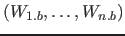: Vector of the to set values
•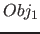: The subobject, for which the variables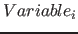are defined and which will be evalued for every variable assignment.

The matrix element represents a matrix withdimensions, which elements are sets ofvalues.

In the matrix element each dimension / counter / index variable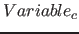(with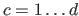) goes through all integers of the corresponding areato. For each integer value of the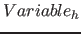all integer values of the variablewill be set. For each value allocation of the dimension variablesthe value variables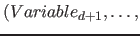will be set to the next set of values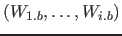. This continues until either the dimension variableshave gone through all of their values or there is no next set of values. If an elementis a variable, so the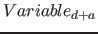will be assigned according to the value of the variable.

If there are no value variables (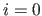), just all the values of the dimension variableswill be set and the sets of values will be ignored.

In listing 1 the operation of the matrix element is shown with C-pseudo code.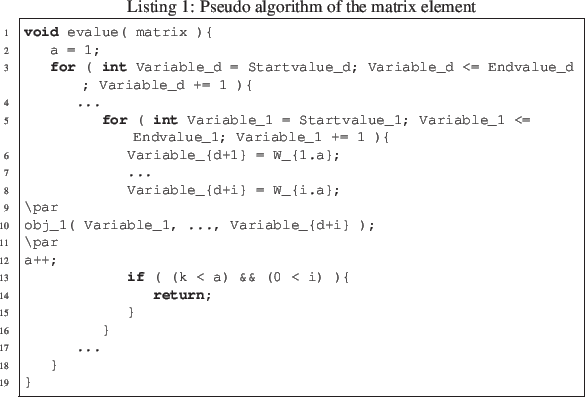Example:

•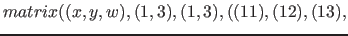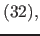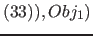: In this example the variables,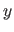and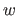will be set for the subobject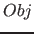sequentially to the values: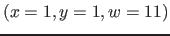,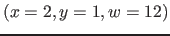,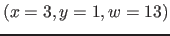,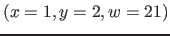,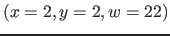,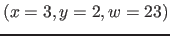,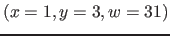,,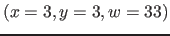•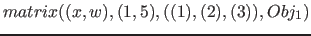: In this example the variablesandwill be set sequentially to the values: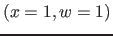,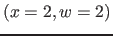,end, because no other assignments forexists
•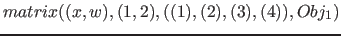: The variablesandwill be set sequentially to the values:,end, because no other assignments forexists to be set
•: The variablesandwill be set sequentially to the values: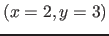,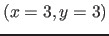,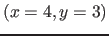,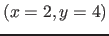,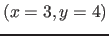,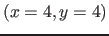Note: Matrices and vectors (one-dimensional matrices) are often used in mathematics, computer science, image processing. With the matrix element for example subimages can directly be specified as a raster image or the values of a flow chart can be given directly.Next: The root-element Up: Elements of the Fib Previous: Set-element   Contents   Index
Betti Österholz 2013-02-13